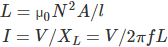# MCQs on Electromagnetic Fields

##### Page 15 of 20. Go to page 1 2 3 4 5 6 7 8 9 10 11 12 13 14 15 16 17 18 19 20
01․ Magnetic field intensity at centre of circular coil, of diameter 1 m and carrying a current of 2 A is
1 A/m.
2 A/m.
4 A/m.
8 A/m.

B = (μ0 I)/2R Magnetic field intensity at centre of circular coil = I/2R.

02․ A rectangular loop in the x – z plane bounded by the lines x = 0, x = a, z = 0 and z = b is in a time varying magnetic field is given by B = B0 cosωt + ay. Where B0 is constant, ω is angular frequency and ay unit vector in the y direction. Now the emf induced in the loop is given by
Zero.
ab Bo cosω t.
abω Bo sinω t.
Bo ω sinω t.

As B = Bo cosω + ay The emf inducted, e(+) = d/dt (B) = ab ω Bo sinω t.

03․ Which of this statement is not characteristic of a static magnetic field
it is conservative.
it is solenoidal.
it has no sink or sources.
magnetic flux lines are always closed.

Although magnetic field is not conservative, magnetic flux is conserved through closed integral B.ds = 0.

04․ The force on a charge moving with velocity v under the influence of electric and magnetic fields is given by which one of the following
q(E + B × V).
q(E + V × B).
q(H + V × E).
q(E +V × H).

The force is required to do work on the particle and therefore changes the kinetic energy, FE = q.E The force on charge moving with velocity v under the magnetic field, FM = q.V × B. So total force on a charge moving with velocity V under influence of electric and magnetic field is called Lorentz force. The value of Lorentz force is q(E + V × B).

05․ Which is the major factor for determining whether a medium is free space, lossless dielectric or a good conductor
Attenuation constant.
Loss tangent.
Reflector coefficient.
Constitutive parameters.

As tanδ = σ/(ωε).

06․ If the static magnetic flux density is B, then
Curl of B = 0.
Divergence of B = 0.
Curl of B = J.
Divergence of B = J.

For static fields the Maxwell equation in integral and differential are Surface integral of (B.ds) = 0 Hence divergence of (B) = 0.

07․ What is the unit of magnetic charge
Ampere-meter square.
Coulomb.
Ampere meter.
Ampere.

The magnetic charge is current element like Idl, Kds, Jdu, having the unit of ampere meter.

08․ Which of the following statement is correct for divergence of electric and magnetic flux densities?
Both are zero.
It is zero for the electric flux density.
These are zero for static densities but non zero for time varying densities.
It is zero for the magnetic flux density.

From Maxwell’s first equation we get, Divergence of (D) = ρ v Divergence of (E) = ρ v So, divergence of electric field intensity is non zero. From Maxwell’s fourth equation we get, divergence of magnetic field intensity is zero.

09․ An inductor designed with 400 turns coil wound on an iron core of 16 cm2 cross sectional area and with a cut of air gap length of 1 mm. The coil is connected to a 230 V, 50 Hz supply. Neglected coil resistance, core loss, iron reluctance and leakage inductance. Find the current in the inductor.
18.08 A.
9.04 A.
4.56 A.
2.28 A.

Inductance is given as10․ In above question no. 9, the average force on the core to reduce the air gap will be
832.29 N.
1666.22 N.
3332.47 N.
6664.8 N.

Energy stored in inductor is given as, E = (1/2) LI2 Force required to reduce the air gap of length 1 mm is F = E/l = E/1 mm.

<<<1314151617>>>